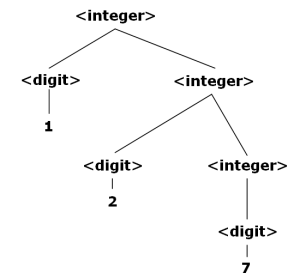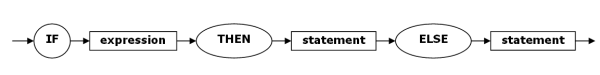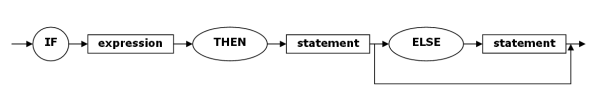# Computer ScienceBackus-Naur Form

In the section on regular expressions, we saw how we could use a regular expression to check if a string conformed to a specific pattern. In one sense, the regular expression defined the 'language' that could be used. The last example on that page shows a regular expression that checks if an email address is in a valid format. This, it could be suggested, is the definition of the 'language' of an email address. Notice that for something as simple as an email address, we have quite a complicated expression,

`[_a-zA-Z\d\-.]+@([_a-zA-Z\d\-]+(\.[_a-zA-Z\d\-]+)+)`

Backus-Naur form is a metasyntactical notation that is used to express the rules for more complex languages. BNF is widely used to describe the grammars of programming languages, protocols and instruction sets.

The following example of BNF describes some of the grammar associated with numbers.

```<digit> ::= 0 | 1 | 2 | 3 | 4 | 5 | 6 | 7 | 8 | 9 <integer> ::= <digit> | <digit> <integer> <fractional number> ::= <integer> | <integer> . <integer>```

The first line defines a digit in the grammar. It can be any of the digits from 0 - 9. We have to specify them all. The second line defines an integer as being one or more digits. This second definition is recursive. An integer is either a single digit or a digit followed by another integer (which could be a single digit or a digit followed by another integer and so on). This removes the need to list all possible combinations. The last line caters for fractional numbers and refers to the definition of an integer.

We could go a lot further in defining how numbers can be written. The point here is to see how the rules for constructing the 'sentences' in this 'language' are listed in BNF.

The expressions inside the <> are called non-terminal symbols. They are the categories of our language - like nouns or verbs. The digits, separated by the pipe character, are the terminal symbols of this grammar. This is, in effect, our alphabet.

We can use the definitions above to see if strings fit the rules for integer and fractional number. The following diagram is called a parse tree. Parse trees describe the way that a given string fits the rules of the grammar. In this case, it shows how the number 127 fits the grammar described above.Grammar rules can also be represented using syntax diagrams. Study the BNF below. This is the format for an IF statement in a high-level programming language.

`<if-statement> ::= IF <expression> THEN <statement> ELSE <statement>`

The syntax diagram for this rule is as follows,Boxes are used for the non-terminals and ovals for the terminals.

Sometimes rules need to be a bit more flexible. In the following BNF, the else statement is shown to be optional with the use of square brackets,

`<if-statement> ::= IF <expression> THEN <statement> [ELSE <statement>]`

In the syntax diagram, the optional part of the expression can be bypassed by following the path that has been added.